Definitions of Square Dance Calls and Concepts
Mix [A1]

Index -->  Plus  |  A1  |  A2  |  C1  |  C2  |  C3A  |  C3B  |  C4  |  NOL  |
Definitions (Text Only) -->  Plus  |  A1  |  A2  |  C1  |  C2  |  C3A  |  C3B  |  C4  |  NOL  |
 Find call:

 \$B8@8l(BFrom any Line of 4 or other applicable formations. EN: 10
\$BG\$0U\$N(B 4 \$B?M\$N(B line \$B\$^\$?\$OB>\$NE,@Z\$J(B formation \$B\$+\$i!%(B JP: 10

1. Centers Cross Run;
2. (new) Centers Trade. EN: 20

A Line of 4 ends in a Line of 4. EN: 30
4 \$B?M\$N(B line \$B\$+\$i\$O(B 4 \$B?M\$N(B line \$B\$G=*\$o\$j\$^\$9!%(B JP: 30

Wave \$B\$+\$i(B: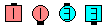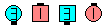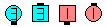Mix\$B\$NA0(B Centers Cross Run\$B\$N8e(B Centers Trade\$B\$N8e(B (\$B=*\$o\$j(B)
One-Faced Line \$B\$+\$i(B: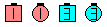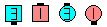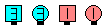Mix\$B\$NA0(B Centers Cross Run\$B\$N8e(B Centers Trade\$B\$N8e(B (\$B=*\$o\$j(B)
T-Bone 2 x 4 \$B\$+\$i(B: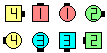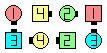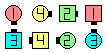Mix\$B\$NA0(B Centers Cross Run\$B\$N8e(B Centers Trade\$B\$N8e(B (\$B=*\$o\$j(B)

\$BCm
• Everyone changes their facing direction by 180°. EN: 40
\$BA40w4i\$N8~\$-\$r(B 180°\$BJQ\$(\$^\$9(B. JP: 40
• Centers should take a slight step forward before doing the Cross Run. This makes the movement smoother for the Ends, as it gives them room to move into the center. EN: 50
Centers \$B\$O(B, \$B>/\$7A0?J\$7\$F\$+\$i(B Cross Run \$B\$r\$9\$Y\$-\$G\$9!%(B \$B\$3\$l\$O(B ends \$B\$,(B center \$B\$NJ}\$X2#\$KF~\$k>l=j\$r\$"\$1\$F(B, \$BF0\$-\$d\$9\$/\$9\$k\$?\$a\$G\$9!%(B update JP: 50
• If both Centers start facing the same direction, they pass Left-shoulders with each other since they are doing a Centers Cross Run (Half Sashay and Run). EN: 60
\$B\$b\$7(B centers \$B\$,F1\$8J}\$r8~\$\$\$F\$\$\$k\$H\$3\$m\$+\$i;O\$a\$k\$H\$-\$O(B, centers \$B\$O\$*8_\$\$\$K(B \$B:88*(B \$B\$GDL\$j2a\$.\$^\$9!%(BCenters \$B\$O(B Cross Run (Half Sashay and Run) \$B\$r\$9\$k\$+\$i\$G\$9!%(B JP: 60
• The original Ends should move into the center without changing their facing direction and then do the Trade. Do not change your facing direction as you move in, as it may confuse others, and make it difficult to properly do the Trade. The confusion occurs because the other End might not be aware of your original facing direction, and hence would not know whether to do an Arm Turn 1/2 (from a Mini-Wave) or a Partner Trade (from a Couple). EN: 70
\$B%*%j%8%J%k\$N(B ends \$B\$O(B \$B8~\$-\$rJQ\$(\$:\$K(B centers \$B\$XF~\$j(B Trade \$B\$r\$9\$Y\$-\$G\$9!%(B \$BF~\$k\$H\$-\$K8~\$-\$rJQ\$(\$F\$7\$^\$&\$H(B, \$B\$b\$&(B1\$B?M\$r:.Mp\$5\$;\$F\$7\$^\$\$(B Trade \$B\$,\$-\$A\$s\$H\$G\$-\$J\$/\$J\$j\$^\$9!%(B \$B\$b\$&(B1\$B?M\$N(B ends \$B\$K\$H\$C\$F(B, \$B\$"\$J\$?\$N%*%j%8%J%k\$N8~\$-\$,J,\$+\$i\$J\$/\$J\$j(B, mini wave \$B\$+\$i(B Arm Turn 1/2 \$B\$r\$9\$k\$N\$+(B, \$B%+%C%W%k\$+\$i(B Partner Trade \$B\$r\$9\$k\$N\$+\$,J,\$+\$i\$J\$/\$J\$j:.Mp\$7\$^\$9!%(B JP: 70
•  Is Mix legal from a Diamond? EN: 80 Mix \$B\$O(B Diamond \$B\$+\$i\$G\$-\$^\$9\$+(B? JP: 80 No. The Centers can not do a Cross Run because there is no one to run around (Cross Run requires that those doing the Run action end in a spot originally occupied by a real dancer). EN: 90 \$B\$\$\$\$\$(!%(BCenters \$B\$K\$H\$C\$F2s\$kAjl=j\$K\$O(B \$B@8?H\$N%@%s%5!<(B \$B\$,\$\$\$J\$1\$l\$P\$J\$j\$^\$;\$s(B)\$B!%(B JP: 90 At C1, the call EN: 100 C1 \$B\$G\$O(B, JP: 100 Squeeze The Diamond [Centers Trade & Spread; then Original Ends slide together & Trade] could be used instead to obtain the desired result of a Mix from a Diamond. EN: 105 [Centers \$B\$O(B Trade & Spread; \$B\$=\$7\$F%*%j%8%J%k\$N(B Ends \$B\$,6aIU\$\$\$F(B Trade] \$B\$,(B, diamond \$B\$+\$i\$N(B Mix \$B\$GK>\$^\$l\$?7k2L\$rF@\$k\$?\$a\$NBe\$o\$j\$H\$7\$F;H\$(\$k\$G\$7\$g\$&!%(B JP: 105

Swing & Mix [A2] (Lee Kopman 1967):
From Facing Couples, a Wave, or an Inverted Line. Arm Turn 1/2; Centers Cross Run; (new) Centers Trade. A Wave or Facing Couples ends in a Wave; an Inverted Line ends in an Inverted Line. This is a 3-part call. EN: 13
\$B8~\$+\$\$9g\$C\$?%+%C%W%k(B, wave, inverted line \$B\$+\$i(B, Arm Turn 1/2 - Centers Cross Run - (new) Centers Trade \$B\$r\$7\$^\$9!%(B Wave \$B\$^\$?\$O8~\$+\$\$9g\$C\$?%+%C%W%k\$+\$i\$O(B wave \$B\$G=*\$o\$j(B, inverted line \$B\$+\$i\$O(B inverted line \$B\$G=*\$o\$j\$^\$9!%(B 3 \$B%Q!<%H\$N%3!<%k\$G\$9!%(B JP: 13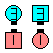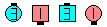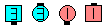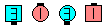Swing & Mix\$B\$NA0(B Arm Turn 1/2\$B\$N8e(B Centers Cross Run\$B\$N8e(B (new) EN: 107 AddJapanese Centers Trade\$B\$N8e(B (\$B=*\$o\$j(B)

\$BCm
• Swing & Mix was the original call, and was later generalized to be anything & MixEN: 110
\$B\$=\$7\$F8e\$K(B anything & Mix \$B\$H0lHL2=\$5\$l\$^\$7\$?!%(B JP: 110
• Swing & Mix is no longer explicitly listed on the CALLERLAB A2 list. However, the calls Swing [A2] and Mix [A1] are on CALLERLAB lists. In our opinion, Swing & Mix should be listed on the A2 list as a separate call because it can be done from Facing Couples. According to the current CALLERLAB definitions, a Swing & Mix is a Swing followed by a Mix. Swing, as defined by CALLERLAB, must start from a Wave or Inverted Line, not Facing Couples. Swing is defined as Ends and Adjacent Centers Arm Turn 1/2. However, Swing & Mix is still widely called from Facing Couples, and it should be legal. EN: 120
• Swing & Mix is a 3-part call due to historical reasons. EN: 130
Swing & Mix \$B\$O(B, \$BNr;KE*\$JM}M3\$K\$h\$j(B 3 \$B%Q!<%H\$N%3!<%k\$G\$9!%(B JP: 130
• 1/4 (or 3/4) Mix [C3A]:
From a R-H Mini-Wave Box, a Diamond with the Centers in a R-H Mini-Wave, or other applicable formations. Those who can Right Arm Turn 1/4 (or 3/4); those in a Line|Wave Mix. Ends in a Line or Wave. This is a 3-part call. EN: 14
\$B1&\$N(B mini wave box, centers \$B\$,1&\$N(B mini wave \$B\$N(B diamond, \$B\$^\$?\$OB>\$NE,@Z\$J(B formation \$B\$+\$i!%(B \$B\$G\$-\$k?M\$,(B Right Arm Turn 1/4 (\$B\$^\$?\$O(B 3/4) \$B\$r\$7(B, line|wave \$B\$G(B Mix \$B\$r\$7\$^\$9!%(B Line \$B\$^\$?\$O(B wave \$B\$G=*\$o\$j\$^\$9!%(B3 \$B%Q!<%H\$N%3!<%k\$G\$9!%(B JP: 14

Grand Mix [C3A]:
From a Generalized Tidal Line. Center pairs of dancers (3 pairs from an 8-dancer Tidal Line, 2 pairs from a 6-dancer Tidal Line) Grand (Working As Centers) Cross Run (like a Grand Slip-Slide); new Center pairs of dancers Trade (Grand Slip). Ends in a Tidal Line. This is a 2-part call. EN: 16
\$B0lHLE*\$J(B tidal line \$B\$+\$i!%(B Center \$B\$N%Z%!(B (8\$B?M(B \$B\$N(B tidal line \$B\$+\$i\$J\$i(B3\$B%Z%!(B, 6\$B?M(B \$B\$N(B tidal line \$B\$+\$i\$J\$i(B2\$B%Z%!(B) \$B\$,(B, Grand (Working As Centers) Cross Run (Grand Slip-Slide \$B\$N\$h\$&\$K(B) \$B\$r\$7(B, \$B?7\$7\$\$(B center \$B\$N%Z%!\$,(B Trade (Grand Slip) \$B\$r\$7\$^\$9!%(B Tidal line \$B\$G=*\$o\$j\$^\$9!%(B 2 \$B%Q!<%H\$N%3!<%k\$G\$9!%(B JP: 16Choreography for MixComments? Questions? Suggestions?

https://www.ceder.net/def/mix.php?level=master&language=japan&action=edit
23-January-2020 21:03:31